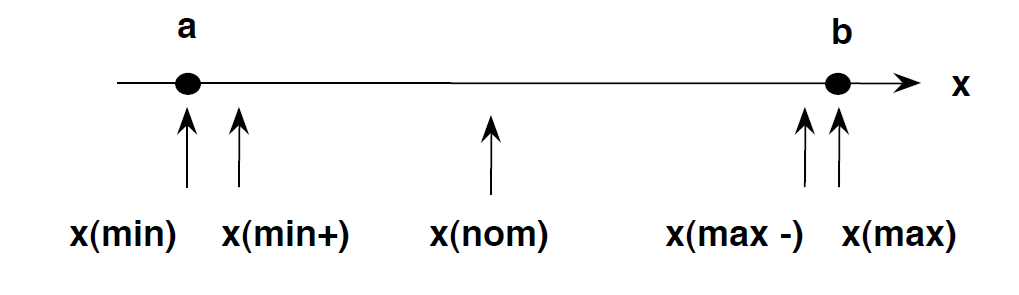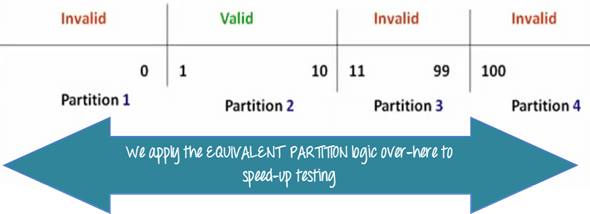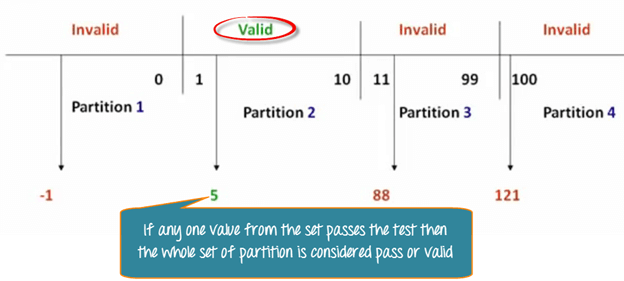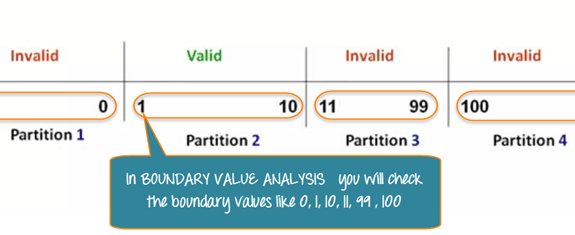Practically, due to time and budget considerations, it is not possible to perform exhausting testing for each set of test data, especially when there is a large pool of input combinations.

• We need an easy way or special techniques that can select test cases intelligently from the pool of test-case, such that all test scenarios are covered.
• We use two techniques - Equivalence Partitioning & Boundary Value Analysis testing techniques to achieve this.

In this tutorial, we will learn

### What is Boundary Testing?

Boundary testing is the process of testing between extreme ends or boundaries between partitions of the input values.

• So these extreme ends like Start- End, Lower- Upper, Maximum-Minimum, Just Inside-Just Outside values are called boundary values and the testing is called "boundary testing".
• The basic idea in boundary value testing is to select input variable values at their:
1. Minimum
2. Just above the minimum
3. A nominal value
4. Just below the maximum
5. Maximum• In Boundary Testing, Equivalence Class Partitioning plays a good role
• Boundary Testing comes after the Equivalence Class Partitioning.

## Equivalence Partitioning

Equivalence Partitioning or Equivalence Class Partitioning is type of black box testing technique which can be applied to all levels of software testing like unit, integration, system, etc. In this technique, input data units are divided into equivalent partitions that can be used to derive test cases which reduces time required for testing because of small number of test cases.

• It divides the input data of software into different equivalence data classes.
• You can apply this technique, where there is a range in the input field.

### Example 1: Equivalence and Boundary Value

• Let's consider the behavior of Order Pizza Text Box Below
• Pizza values 1 to 10 is considered valid. A success message is shown.
• While value 11 to 99 are considered invalid for order and an error message will appear, "Only 10 Pizza can be ordered"
Order Pizza:

Here is the test condition

1. Any Number greater than 10 entered in the Order Pizza field(let say 11) is considered invalid.
2. Any Number less than 1 that is 0 or below, then it is considered invalid.
3. Numbers 1 to 10 are considered valid
4. Any 3 Digit Number say -100 is invalid.

We cannot test all the possible values because if done, the number of test cases will be more than 100. To address this problem, we use equivalence partitioning hypothesis where we divide the possible values of tickets into groups or sets as shown below where the system behavior can be considered the same.The divided sets are called Equivalence Partitions or Equivalence Classes. Then we pick only one value from each partition for testing. The hypothesis behind this technique is that if one condition/value in a partition passes all others will also pass. Likewise, if one condition in a partition fails, all other conditions in that partition will fail.Boundary Value Analysis- in Boundary Value Analysis, you test boundaries between equivalence partitionsIn our earlier example instead of checking, one value for each partition you will check the values at the partitions like 0, 1, 10, 11 and so on. As you may observe, you test values at both valid and invalid boundaries. Boundary Value Analysis is also called range checking.

Equivalence partitioning and boundary value analysis(BVA) are closely related and can be used together at all levels of testing.

### Example 2: Equivalence and Boundary Value

Following password field accepts minimum 6 characters and maximum 10 characters

That means results for values in partitions 0-5, 6-10, 11-14 should be equivalent

Test Scenario # Test Scenario Description Expected Outcome
1 Enter 0 to 5 characters in password field System should not accept
2 Enter 6 to 10 characters in password field System should accept
3 Enter 11 to 14 character in password field System should not accept

### Examples 3: Input Box should accept the Number 1 to 10

Here we will see the Boundary Value Test Cases

 Test Scenario Description Expected Outcome Boundary Value = 0 System should NOT accept Boundary Value = 1 System should accept Boundary Value = 2 System should accept Boundary Value = 9 System should accept Boundary Value = 10 System should accept Boundary Value = 11 System should NOT accept

### Why Equivalence & Boundary Analysis Testing

1. This testing is used to reduce a very large number of test cases to manageable chunks.
2. Very clear guidelines on determining test cases without compromising on the effectiveness of testing.
3. Appropriate for calculation-intensive applications with a large number of variables/inputs

Summary:

• Boundary Analysis testing is used when practically it is impossible to test a large pool of test cases individually
• Two techniques - Equivalence Partitioning & Boundary Value Analysis testing techniques are used
• In Equivalence Partitioning, first, you divide a set of test condition into a partition that can be considered.
• In Boundary Value Analysis you then test boundaries between equivalence partitions
• Appropriate for calculation-intensive applications with variables that represent physical quantities

YOU MIGHT LIKE: